# ACT Math Practice Test 7

Difficulty Level – 4: Difficult

Directions: Solve each problem and then click on the correct answer. You are permitted to use a calculator on this test.

Congratulations - you have completed . You scored %%SCORE%% out of %%TOTAL%%. Your performance has been rated as %%RATING%%
 Question 1
In a competition where the highest score wins, the scores of the top four competitors are consecutive even integers whose sum is 36. What is the score of the competitor who finished in second place?

 A 6 B 8 C 10 D 12 E 14
Question 1 Explanation:
The correct answer is (C). Use an equation to represent the situation, x + x + 2 + x + 4 + x + 6 = 36. Solve for x to find 6, but recognize that this is the least of the four scores. The score of the competitor who finished in second place will be x + 4 = 6 + 4 = 10.
 Question 2
A company will be using a pie chart to represent the total expenditures of its various departments. If the breakdown of its expenditures is as follows: 19% Research and Development, 33% Marketing, 22% Payroll. If the remaining expenditures represent \$12,250, what is the dollar amount spent on Research and Development (Round to nearest unit)?

 A 7750 B 7927 C 8331 D 8952 E 9113
Question 2 Explanation:
The correct answer is (D). Because we are presented with a pie chart, we know the percentages spent on each department will add up to 100%, meaning the remaining expenditures represent 26% of all expenditures. We can set up a proportion between the 19% spent on R&D and the 26% spent on others. 19/26 = x/12,250. Cross multiply to solve for x to find 8951.9 which rounds to 8952.
 Question 3The vertices of square ABCD have coordinates (0,0), (-8,0), (-8,8), and (0,8) in the standard (x, y) coordinate plane, and circle O is inscribed in square ABCD. Which of the following equations represents circle O?

 A (x + 4)2 + (y + 4)2 = 4 B (x − 4)2 + (y − 4)2 = 16 C (x + 4)2 + (y − 4)2 = 16 D (x + 4)2 + (y − 4)2 = 4 E (x − 4)2 + (y + 4)2 = 8
Question 3 Explanation:
The correct answer is (C). The equation of a circle is given in the form (xa)2 + (yb)2 = r2, where a is the x-coordinate of the center point, b is the y-coordinate of the center point, and r is the radius. Since square ABCD has sides of length 8, we know that circle O must have a radius of 4 units. Therefore, the equation of circle O should end in the term 42 or 16. We can eliminate answers (A), (D), and (E), because they do not meet this condition.

The center of circle O has an x-coordinate of −4, so we would expect circle O's equation to contain the term (x − (−4))2, or (x + 4)2. This eliminates answer (B) from consideration, leaving us with answer (C) as the correct answer. Since circle O has center (−4, 4) and radius 4, its full equation should read (x + 4)2 + (y − 4)2 = 16.
 Question 4
The average of 7 numbers is 24. The smallest of the numbers is 2 and the largest of the numbers is 31. What is the average of the middle 5 numbers?

 A 25 B 27 C 30 D 31 E 32
Question 4 Explanation:
The correct answer is (B). Begin by setting up an equation representing the average. (2 + x + 31) ÷ 7 = 24. Solve for x to find 135 and recognize that this x represents the sum of the remaining 5 scores. To find the average, divide 135 by 5 to find 27.
 Question 5
Two side lengths of a triangle are 5 and 6, which of the following CANNOT be the length of the third side?

 A 2 B 3 C 5 D 10 E 12
Question 5 Explanation:
The correct answer is (E). If a triangle has side lengths a, b, and c, the sum of the lengths of any 2 sides must be larger than the length of the 3rd side. So in this case 5 + 6 = 11 must be larger than side length c. From the answer choices, 12 is the only length greater than 11, so it cannot be the length of the third side.
 Question 6
Danny wishes to shift the graph of the function ƒ(x) = x² four places to the right and six places down from the origin (0,0). Which equation represents this translation?

 A ƒ(x) = (x − 6)2 − 6 B ƒ(x) = x − 42 + 6 C ƒ(x) = x + 42 − 6 D ƒ(x) = (x2 − 4)2 − 6 E ƒ(x) = (x − 4)2 − 6
Question 6 Explanation:
The correct answer is (E). Recall that vertex- form of a parabola is:
a(xh)2 + k, where (h, k) represents the vertex.

We wish to translate our vertex from (0,0) to (4,−6) so h = 4 and k = −6.
ƒ(x) = (x − 4)2 − 6
 Question 7
The following input and output values represent a linear relationship. What number does the variable j represent?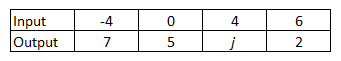A 2 B 3 C 4 D 5 E 6
Question 7 Explanation:
The correct answer is (B). If the numbers represent a functional relationship, then the slope of the line formed by each 2 points will be the same: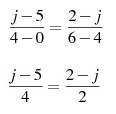Cross multiply and solve for j:
2j − 10 = 8 − 4j
6j = 18
j = 3
 Question 8
What is the matrix product of: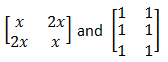A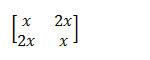B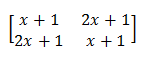C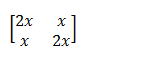D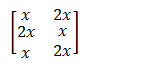E Cannot be determined.
Question 8 Explanation:
The correct answer is (E). Here we are given a 2x2 matrix on the left, and a 3x2 matrix on the right. You may recall that in order to find the product of two matrices, the number of columns in the first matrix must equal the number of rows in the second matrix. For example, a 3x1 matrix multiplied with a 1x3 matrix results in a 3x3 matrix. If the number of columns in the first matrix doesn’t match the number of rows in the second column, in this question for example, we are unable to find the product of the matrices. Consequently, answer choice (E) is correct.
 Question 9
Given that logxa = 2 and logxb = 3, what is the value of logx(ab)3?

 A 6 B 15 C 36 D 54 E 216
Question 9 Explanation:
The correct answer is (B). The term we are interested in, logx(ab)3, is equivalent to 3logx(ab).

This can also be expressed as 3logxa + 3logxb, and since we know the values of logxa and logxb, we can substitute to find the answer:

logx(ab)3
= 3logxa + 3logxb
= 3(2) + 3(3)
= 15 (Choice B).

If you don't know these logarithmic identities, you can still solve the problem by finding values for x, a, and b that satisfy the conditions. Then, simply calculate the value of log(x)(ab)3. The easiest way to do this is to work with a base of 10, which would mean that x = 10, a = 100, and b = 1,000. We can then calculate the answer:

logx(ab)3
= log(10)(100 * 1,000)3
= log(10)(1,000,000,000,000,000)
= log(10)(1015)
= 15
 Question 10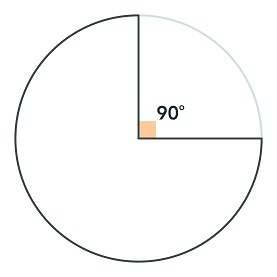There is to be a walkway built around a circular pond. The walkway will include a curved section extending most of the way around the pond and two straight sections, each measuring 15 feet, extending from the edges that meet in the center at a 90 degree angle to each other, as shown in the figure above. Which of the following is closest to the total length of the walkway, in feet?

 A 30 B 65 C 71 D 101 E 131
Question 10 Explanation:
The correct answer is (D). The curved section extends all the way around the edge of the pond except the part subtended by the 90-degree angle; therefore, its length is ¾ of the perimeter of the circle, or (¾) (2*π*r). The radius is 15.

(¾)(30π)

22.5π = length of the curved portion of the walkway. Pi is about 3, so we can estimate this as 67.5.

Each of the straight segments has length equal to the radius of the pond, or 15 feet, so the total length is around 67.5 + 15 + 15 = 97.5. The actual length will be a little greater than 97.5. The closest answer choice is (D) 101.
Once you are finished, click the button below. Any items you have not completed will be marked incorrect.
There are 10 questions to complete.
 ← List →